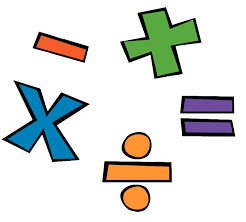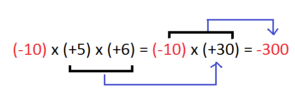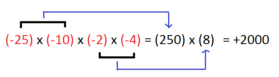Courses

# Operations on Integer Numbers Class 7 Notes | EduRev

## Class 7 : Operations on Integer Numbers Class 7 Notes | EduRev

The document Operations on Integer Numbers Class 7 Notes | EduRev is a part of the Class 7 Course Mathematics (Maths) Class 7.
All you need of Class 7 at this link: Class 7

Integer Numbers and Mathematical Operations
Mathematical operations include addition, subtraction, multiplication, and division of any number. When we perform these operations with integer numbers we always keep in mind the sign before every number.

As we already know that an integer includes a number with a positive or negative sign, therefore, these have to be dealt with different perceptions. Before delving into further operations, we first need to know the properties related to these mathematical operations.

Addition and Subtraction of Integer numbersProperty of Closure in Addition and Subtraction
When we add two whole numbers, the answer is a whole number. This is called the closure property of additions. Now, do integers also follow this property? Let’s see:
10 + (-12) = -2
Is -2 an integer number. Since it has a negative sign with it, therefore we call it an integer number. From the above example, we can say that the sum of two integers is also an integer. So when an integer a is added with another integer b the answer is always an integer.
Let’s take some more examples to support our observation:

• 19+10 = +29
• 15 + (-20) = -5

In both the examples, the solution is an integer. Therefore every integer when summed up with an integer gives an integer. What happens when an integer is subtracted from an integer. Let’s see the following examples:

• 10- 3 = +7
• 15 – (-7) = -22

In both the examples, the difference between two integers gives an integer in solution. This means that integers are also closed under subtraction. Hence, in the case of subtraction of two integers a and b the answer to a-b is also an integer.

Commutative Property
Commutative property states the following:
a+b = b+a
For whole numbers, we already know that the sum of two whole numbers is always the same. But is the case true for integer numbers as well? The sum of two integer numbers is also, always the same. This means that integer numbers also follow the commutative property like whole numbers. Let’s see the following examples:

• 15 + 20 = 35; 20 +15 = 35
• -10 + (-5) = -15; -5 + (-10) = -15

The above examples prove that the addition of integers is commutative. Is the case true with subtractions? Are subtractions also commutative? The following examples will let us know this:

• 5-(-3) = +8
• -3-5 = -8

Are the integer numbers same? The answer is a no! This brings us to a conclusion that subtractions of integers are not commutative. Therefore, a-b ≠ b-a

Associative Property
According to associative property of whole numbers a,b,c,

• [a+b]+c = a+[b+c]

Does this property also apply to integer numbers? Let’s see:

• [(-4)+(-6)]+(-2) = -12
• (-4) + [(-6)+(-2)] = -12

The answer in both the cases is the same. So the sum of integer numbers abides the associative property of addition.

The additive identity of any number is checked with the help of zero. For all the whole numbers, zero proves to be their additive identity. This means that when a whole number is added with a zero, the answer is the whole number itself. Is the case same with integer numbers as wel?. The answer here is a yes. The following examples prove our observation:

• +10+0 = +10
• -9+0 = -9

Therefore for any integer, x,
x+0 = x = 0+x

Multiplication of Integers
After addition and subtraction, we need to understand the multiplication of two integer numbers. Let’s look at some of its properties.

Closure Property
Is the product of two integer numbers also an integer number? Well, yes the product of every integer is an integer.

Commutative Property
The product of two same integers is always the same. This means that
a × b = b× a

Multiplication with Zero
Every integer, when multiplied with a zero, gives zero as the answer.
a × 0 = 0

Multiplicative Identity
Every integer number, when multiplied with 1, gives an integer number in an answer.

• a×1 = a
• (-a)×1 = -a

Associative Property
Integer numbers also abide by the associative property of multiplication. This implies that like whole numbers the grouping of integers does not affect the product of integers. Thus,
(a×b)×c = (a)×(b×c)

Distributive Property
Here we shall check the distributivity of integers over addition is true or not.

• (-4) × (2 + 6) = -32
• (-4) × (2) + (-4) × (+6) = (-8) +(-24) = -32

The example above shows that multiplication of integers also shows distributivity of multiplication over addition. Thus,
a × (b+c) = (a×b) + (a×c)
Does the distributive property also comply with subtractions in multiplication? The answer is a yes! The example below will help you understand:

• (-8) × (5 – 3) = -16
• [(-8) ×(+ 5)] – [(-8) × (-3)] =( -40)- (+24) = -16

From the above example we can thus state that;
a × (b-c) = (a×b) – (a×c)

Multiplication of a Negative and Positive Integer
As already said earlier, while performing the mathematical operations of integer numbers, we must always keep in mind the respective sign before every number. When multiplying two integers with a negative and positive sign, the product shall always be negative.

This means that in every case of multiplication between contradictorily signed integers the answer shall always have a negative sign. For example:
4 ×(-5) = -20
To simplify the product we write the above example as :
(-5)+(-5)+(-5)+(-5) = -20
Some more examples are:

• -3×4 = -12
• 12×-6 = -72

Therefore  (-a) × b = a × (– b) = – (a × b)
The multiplication of integers is similar to multiplication of whole numbers. The only difference here is that after multiplying we put a minus sign (-) in the answer.

Multiplication of Two Negative Integers
The multiplication of two negative integer numbers is always a positive integer. This means the product of (-a) ×(-b) = +c. The negative integer numbers in multiplication are multiplied like ordinary whole numbers. Some examples will help you understand this in a better way:

• (-3) × (-6) = 18
• (-30)×(-15) = 450

This means that: (-a) ×(-b) = a×b. The minus (-) sign of the integer numbers while multiplication is ignored.

Product of Three or More Integers
In the above topics, we multiplied only two integers. Now we shall multiply three or more than three integers. Consider the following image:In the above set of examples, what do you notice? When we multiply more than three integers, we multiply them in the set of two. The signs of the answer depend on the rules we already mentioned in the multiplication of positive and negative integer.In both the example images we notice that when we multiply two negative integers the answer is a positive integer. When we multiply two integers with negative and positive signs respectively, the answer is a negative integer number.

In case you are confused about signs, you may follow another very simple way of applying a sign. Now, if the number of negatively signed numbers is odd, the answer shall be a negative integer. If the negative integers are in even number then the answer shall be a positive integer.
(-a) × (-b) × (-c) × (-d) = +z
In the example given above, the total number of integers are even in number, hence the answer is a positive integer.
(-a) × (-b) × (-c) × (-d) × (-e) = -z
See the example given above. The total number of integers are odd in number, hence answer is a negative integer.

Division of Integer numbers
We know that divisions are inverse of multiplications. How do we then perform divisions in integers? Division of integers is similar to the division of whole numbers. While dividing an integer with an integer we divide them like normal whole numbers and then put the sign respective to the integers involved.

This means that when we divide a positive integer with a positive integer the answer is a positive integer. Similarly, when the division involves one positive and one negative integer then, we divide them like normal whole numbers and put a negative sign in the quotient. For example:

• 72÷(–8) = -9
• (-80)÷(5) = -16

Now, let’s look at some of the properties for the division of integers.

Closure Property of Division
Is the division of two integer numbers also an integer number? Well, no. The division of two numbers does not give an integer in the answer.

• (-8)÷(2) = -4
• (-9)÷(2) = -9/2

So the division of integers does not give an integer in the answer.

Commutative Property of Division
This property does not apply to divisions between integers. This means that a÷b ≠b÷a

Division by Zero
Like whole numbers, an integer number also cannot be divided by (zero) 0, but when zero (0) is divided by an integer the answer is zero (0).

Division by One
Every integer number, when divided by 1, gives the same integer number in the answer. But the case is not the same when an integer is divided by -1

• a÷1 = a
• (a)÷(-1) = -a

From the above illustrations, we have come to know that the mathematical operations of integer number involve cautious calculations as these follow a different set of rules.

Solved Examples
Ques: In a test (+5) marks are given for every correct answer and (–2) marks are given for every incorrect answer.

1. Shyam answered all the questions and scored 40 marks though he got 10 correct answers.
2. Renu also answered all the questions and scored (–16) marks though he got only 4 correct answers.

Sol: One correct answer gives = +5 marks.
Shyam gave 10 correct answers, so, marks given for 10 correct answers = 5 × 10 = 50. Shyam’s score = 40.
Marks obtained for incorrect answers = 40 – 50 = – 10
Marks given for one incorrect answer = (–2)
Therefore, number of incorrect answers = (–10) ÷ (–2) =5
Marks given for 4 correct answers = 5 × 4 = 20
Renu’s score = –16
Marks obtained for incorrect answers = –16 – 20 = – 36
Marks given for one incorrect answer = (–2)
Therefore number of incorrect answers = (–36) ÷ (–2) = 18

Offer running on EduRev: Apply code STAYHOME200 to get INR 200 off on our premium plan EduRev Infinity!

,

,

,

,

,

,

,

,

,

,

,

,

,

,

,

,

,

,

,

,

,

;